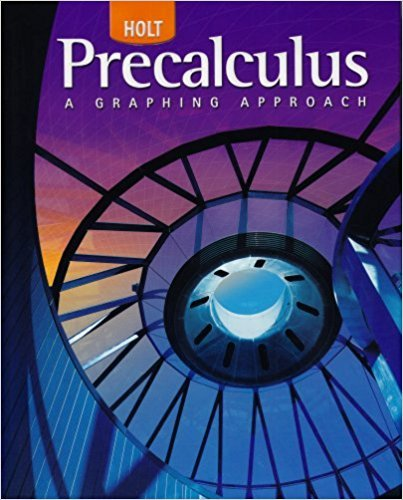×
×

# Solutions for Chapter 12.3: Systems and Matrices## Full solutions for Precalculus | 1st Edition

ISBN: 9780030416477Solutions for Chapter 12.3: Systems and Matrices

Solutions for Chapter 12.3
4 5 0 312 Reviews
17
2
##### ISBN: 9780030416477

Precalculus was written by and is associated to the ISBN: 9780030416477. Chapter 12.3: Systems and Matrices includes 8 full step-by-step solutions. Since 8 problems in chapter 12.3: Systems and Matrices have been answered, more than 26196 students have viewed full step-by-step solutions from this chapter. This textbook survival guide was created for the textbook: Precalculus, edition: 1. This expansive textbook survival guide covers the following chapters and their solutions.

Key Calculus Terms and definitions covered in this textbook
• Central angle

An angle whose vertex is the center of a circle

• Compounded monthly

See Compounded k times per year.

• Differentiable at x = a

ƒ'(a) exists

• Divergence

A sequence or series diverges if it does not converge

• Domain of validity of an identity

The set of values of the variable for which both sides of the identity are defined

• Equilibrium point

A point where the supply curve and demand curve intersect. The corresponding price is the equilibrium price.

• Factor

In algebra, a quantity being multiplied in a product. In statistics, a potential explanatory variable under study in an experiment, .

• Five-number summary

The minimum, first quartile, median, third quartile, and maximum of a data set.

• Function

A relation that associates each value in the domain with exactly one value in the range.

• Horizontal Line Test

A test for determining whether the inverse of a relation is a function.

• Imaginary part of a complex number

See Complex number.

• Increasing on an interval

A function ƒ is increasing on an interval I if, for any two points in I, a positive change in x results in a positive change in.

• Jump discontinuity at x a

limx:a - ƒ1x2 and limx:a + ƒ1x2 exist but are not equal

• Periodic function

A function ƒ for which there is a positive number c such that for every value t in the domain of ƒ. The smallest such number c is the period of the function.

• Polar coordinate system

A coordinate system whose ordered pair is based on the directed distance from a central point (the pole) and the angle measured from a ray from the pole (the polar axis)

• Sum of a finite geometric series

Sn = a111 - r n 2 1 - r

A graph in which (-x, -y) is on the the graph whenever (x, y) is; or a graph in which (-r, ?) or (r, ? + ?) is on the graph whenever (r, ?) is

• Term of a polynomial (function)

An expression of the form anxn in a polynomial (function).

• Vertical line test

A test for determining whether a graph is a function.

• Work

The product of a force applied to an object over a given distance W = ƒFƒ ƒAB!ƒ.

×